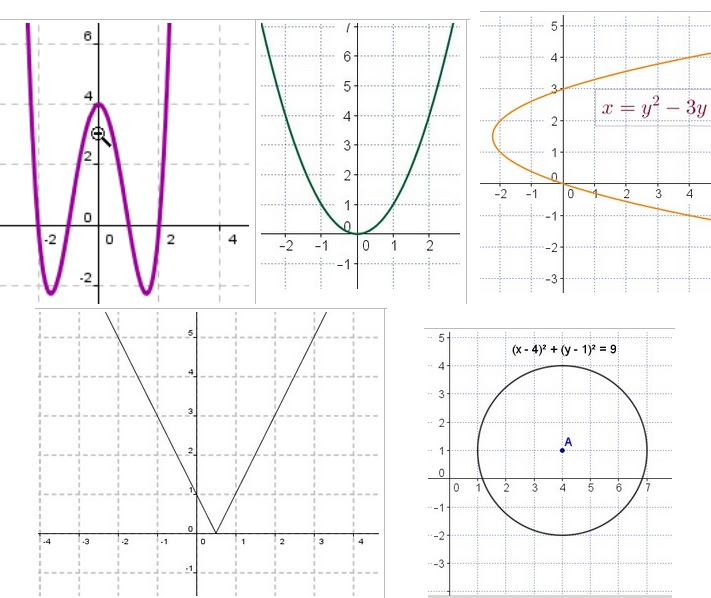# Deciding if a Graph is a Function

Posted on: April 15, 2020 Posted by: Susan Johnsey Comments: 0What determines if a graph is a function? Look for two points (or more is ok) on the graph that have the same x-coordinate, but different y-coordinates. If you canNOT find any then the graph is FUNCTION.

I forgot to give you the answers in first post. They are at the bottom.

RECALL for points there are two numbers ( we are just thinking in 2-dimensions right now!) The numbers are inside parentheses and separated by a comma. The first number is called the x-coordinate and the second number is called the y-coordinate. That’s a little review for you, but I hate to tell there are other names! But most countries do use the (x, y).

If you SEE the points (3, -2) and (3, 5) on your graph then your graph is NOT a function. Every x-coordinate of the graph can have only 1 y-coordinate associated with it. Can you find 2 (or more points) that have the same x but different y? If so, then that is NOT a function.

Look at your graph carefully.  Choose some points that are directly above each other! That means they have the same x-coordinate. If you can then it is NOT a function.  When points are directly above each other then you could imagine a vertical line would go through them. There is a method taught by many teachers that uses a vertical line. Perhaps you have heard of the vertical line test! Would you like a video? Google: Vertical line test for functions.

Look at my 5 graphs, one at a time. Which of my 5 graphs in my diagram are NOT functions? STOP and think. They are the two on the right side. The circle and the parabola that is opening towards the right, “U on its side”.